# 9.1 In-Line Mathematics

In-line mathematics is created using the math environment. (Note U.S. spelling -- “math” not “maths”).

Example:

The variable \begin{math}x\end{math} is transformed by the function \begin{math}f(x)\end{math}.

It's somewhat cumbersome having to type \begin{math} and \end{math} and it also makes the source code a little difficult to read so there are shorthand notations that can be used instead: $$is equivalent to \begin{math} and$$ is equivalent to \end{math}. So the example above can be rewritten:

The variable $$x$$ is transformed by the function $$f(x)$$.

There is an even shorter notation: The special character $is equivalent to both \begin{math} and \end{math}: The variable$x$is transformed by the function$f(x)$. This is considerably easier to type and to read, but you need to make sure that all your$ symbols have matching pairs. The above code will look like: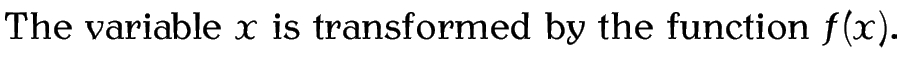The other advantage in using $over $$and$$ is that$ is a robust command, whereas $$and$$ are fragile commands and will need to be protected if they occur in a moving argument.

Note: you should always make sure you are in maths mode to typeset any variables (such as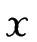,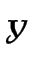,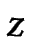), as this will ensure that the correct maths fonts are used, as well as the appropriate spacing. Similarly, don't use $as a short cut for an italic font. Notice the$difference$between$(x', y', z')\$ and \textit{(x', y', z')}.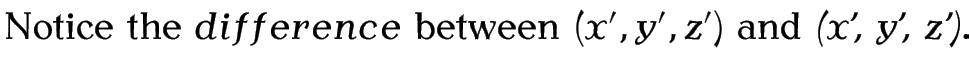This book is also available as A4 PDF or 12.8cm x 9.6cm PDF or paperback (ISBN 978-1-909440-00-5).

© 2012 Dickimaw Books. "Dickimaw", "Dickimaw Books" and the Dickimaw parrot logo are trademarks. The Dickimaw parrot was painted by Magdalene Pritchett.